# Basic Geometry Terminology: Point, Line, Line Segment, Ray, Plane, Plane Figure

To understand geometry you must understand its basic terminology.

Point

A point is an exact location in 2D or 3D space. It has no size, that is, it has no width, length, depth, radius, or diameter. A point is represented by a dot. A point in geometry is usually named using a capital letter.

Line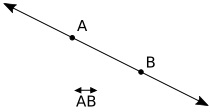A line is straight path of points, it has no curves. It extends infinitely in two directions. A line has one dimension, length. A line is named by naming two points on the line and drawing a double arrowed line over the letters.

Even though the line extends indefinitely in either direction beyond the two points, it can be named using the names of any two points on the line. Lines can also be named with lowercase letters or a single lower case letter.

Line Segment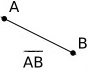If a line has ends it is called a "Line Segment". A Line Segment is named using the capital letters of its beginning point and ending point and drawing a line over the letters. A line segment may also be named using a single letter. By convention, this is usually a lower case letter.

Ray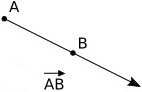If a line has one end, and the other end extends infinitely in one direction, it is a "Ray". The point where the ray starts is called its endpoint. You cannot measure the length of a ray. A ray is named using its endpoint and any other point on the ray.

Plane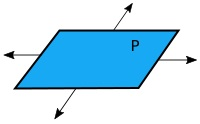A "Plane" is a flat surface that extends infinitely far. A plane is two dimensional. Points on the same plane are said to be coplanar points. A plane is named using a capital letter or it can be named using the names of at least three points on the plane.

Plane Figure

A plane figure is in a plane and is formed by three or more line segments meeting only at their endpoints. A plane figure can be made of straight lines, curved lines, or both straight and curved lines, as long as their line segments lie in the same plane. Different plane shapes are named by their attributes, such as the numbers of sides or corners.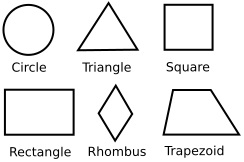Some plane shapes are circle, triangle, square, rectangle, rhombus, and trapezoid. Any simple, closed plane figure formed by three or more line segments meeting only at their endpoints is called a polygon.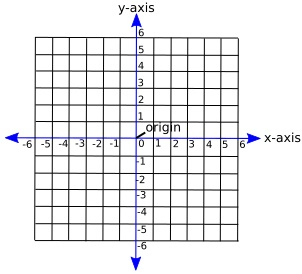In coordinate geometry, points are located on the plane using their coordinates - two numbers that show where the point is positioned with respect to two number line "axes" at right angles to each other. One axis running across the plane is called the "x axis" and another at right angles to it is called the "y axis". The point where the axes cross is called the "origin" and is where both x and y are zero.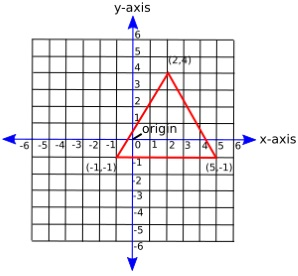In addition to a plane shape being named by its attributes, it can be identified by it's coordinates in the plane. Shown above is the plane figure identified by the coordinates (2.4)(-1,-1)(5,-1).